# Aligned Induction

## MNIST - Model induction

MNIST - handwritten digits/Model induction

We shall analyse this dataset using the NIST repository which depends on the AlignmentRepa repository.

### Sections

Model 1 - 15-fud

Model 2 - 15-fud

Model 3 - All pixels

Model 4 - All pixels

Model 35 - All pixels

Model 34 - Averaged pixels

Model 5 - 15-fud square regions of 10x10 pixels

Model 6 - Square regions of 10x10 pixels

Model 21 - Square regions of 15x15 pixels

Model 10 - Centred square regions of 11x11 pixels

Model 18 - Row and column regions

Model 24 - Two level over 10x10 regions

Model 25 - Two level over 15x15 regions

Model 26 - Two level over centred square regions of 11x11 pixels

NIST test

NIST conditional

Model 36 - Conditional level over 15-fud

Model 37 - Conditional level over all pixels

Model 38 - Conditional level over averaged pixels

NIST test averaged

NIST conditional over square regions

Model 43 - Conditional level over square regions of 15x15 pixels

Model 40 - Conditional level over two level over 10x10 regions

Model 41 - Conditional level over two level over 15x15 regions

Model 42 - Conditional level over two level centred square regions of 11x11 pixels

### Model 1 - 15-fud

Consider a 15-fud induced model of 7500 events of the training sample. We shall run it in the interpreter,

:l NISTDev

(uu,hrtr) <- nistTrainBucketedIO 2

let digit = VarStr "digit"
let vv = uvars uu
let vvl = sgl digit
let vvk = vv minus vvl

let hr = hrev [i | i <- [0.. hrsize hrtr - 1], i mod 8 == 0] hrtr

let hrtr = undefined

let (wmax,lmax,xmax,omax,bmax,mmax,umax,pmax,fmax,mult,seed) = (2^10, 8, 2^10, 10, (10*3), 3, 2^8, 1, 15, 1, 5)

Just (uu1,df) <- decomperIO uu vvk hr wmax lmax xmax omax bmax mmax umax pmax fmax mult seed



This runs in the ghci interpreter on a Ubuntu 16.04 Pentium CPU G2030 @ 3.00GHz in 1333 seconds.

summation mult seed uu1 df hr
(148378.04791361679,74189.02395680839)

card $dfund df 147 card$ fvars $dfff df 535 BL.writeFile ("NIST_model1.json")$ decompFudsPersistentsEncode $decompFudsPersistent df  Imaging the fud decomposition, let file = "/tmp/NIST.bmp" let hrbmav = hrbm 28 3 2$ hr hrhrred vvk

let pp = qqll $treesPaths$ hrmult uu1 df hr

rpln $map (map (hrsize . snd)) pp "[7500,2584,1203]" "[7500,2584,1378,793]" "[7500,4909,1681,687]" "[7500,4909,1681,972]" "[7500,4909,3221,1395,941]" "[7500,4909,3221,1746,628]" "[7500,4909,3221,1746,1118]" bmwrite file$ bmvstack $map (\bm -> bminsert (bmempty ((28*2)+2) (((28*2)+2)*(maximum (map length pp)))) 0 0 bm)$ map (bmhstack . map (\((_,ff),hrs) -> bmborder 1 (bmmax (hrbm 28 2 2 (hrs hrhrred vvk)) 0 0 (hrbm 28 2 2 (qqhr 2 uu vvk (fund ff)))))) pp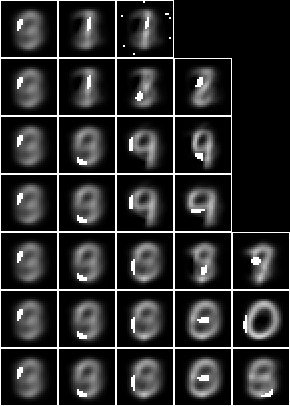Both the averaged slice and the fud underlying are shown in each image to indicate where the attention is during decomposition.

### Model 2 - 15-fud

Now consider a similar model but with a larger shuffle multiplier, mult,

:l NISTDev

(uu,hrtr) <- nistTrainBucketedIO 2

let digit = VarStr "digit"
let vv = uvars uu
let vvl = sgl digit
let vvk = vv minus vvl

let hr = hrev [i | i <- [0.. hrsize hrtr - 1], i mod 8 == 0] hrtr

let hrtr = undefined

let (wmax,lmax,xmax,omax,bmax,mmax,umax,pmax,fmax,mult,seed) = (2^10, 8, 2^10, 10, (10*3), 3, 2^8, 1, 15, 3, 5)

Just (uu1,df) <- decomperIO uu vvk hr wmax lmax xmax omax bmax mmax umax pmax fmax mult seed



This runs in the ghci interpreter on a Ubuntu 16.04 Pentium CPU G2030 @ 3.00GHz in 1785 seconds.

summation mult seed uu1 df hr
(122058.33490991575,59900.18390268337)

card $dfund df 147 card$ fvars $dfff df 542 BL.writeFile ("NIST_model2.json")$ decompFudsPersistentsEncode $decompFudsPersistent df  Imaging the fud decomposition, let pp = qqll$ treesPaths $hrmult uu1 df hr rpln$ map (map (hrsize . snd)) pp
"[7500,2584,584]"
"[7500,2584,1295,532]"
"[7500,2584,1295,749]"
"[7500,4513,1578,591]"
"[7500,4513,1578,964,595]"
"[7500,4513,2633,1154]"
"[7500,4513,2633,1235,764]"

printf "alignment density: %.2f\n" ad hFlush stdout  The statistics are, model cardinality: 3715 selected train size: 7500 alignment: 165090.07 alignment density: 80621.53  Finally the images are written out,  let pp = qqll treesPaths $hrmult uu1 df1 hr' bmwrite (model ++ ".bmp")$ bmvstack $map (\bm -> bminsert (bmempty (28+2) ((28+2)*(maximum (map length pp)))) 0 0 bm)$ map (bmhstack . map (\(_,hrs) -> bmborder 1 (hrbm 28 1 2 (hrs hrhrred vvk)))) $pp bmwrite (model ++ "_1.bmp")$ bmvstack $map (\bm -> bminsert (bmempty ((28*1)+2) (((28*1)+2)*(maximum (map length pp)))) 0 0 bm)$ map (bmhstack . map (\((_,ff),hrs) -> bmborder 1 (bmmax (hrbm 28 1 2 (hrs hrhrred vvk)) 0 0 (hrbm 28 1 2 (qqhr 2 uu vvk (fund ff)))))) $pp bmwrite (model ++ "_2.bmp")$ bmvstack $map (\bm -> bminsert (bmempty ((28*2)+2) (((28*2)+2)*(maximum (map length pp)))) 0 0 bm)$ map (bmhstack . map (\((_,ff),hrs) -> bmborder 1 (bmmax (hrbm 28 2 2 (hrs hrhrred vvk)) 0 0 (hrbm 28 2 2 (qqhr 2 uu vvk (fund ff)))))) pp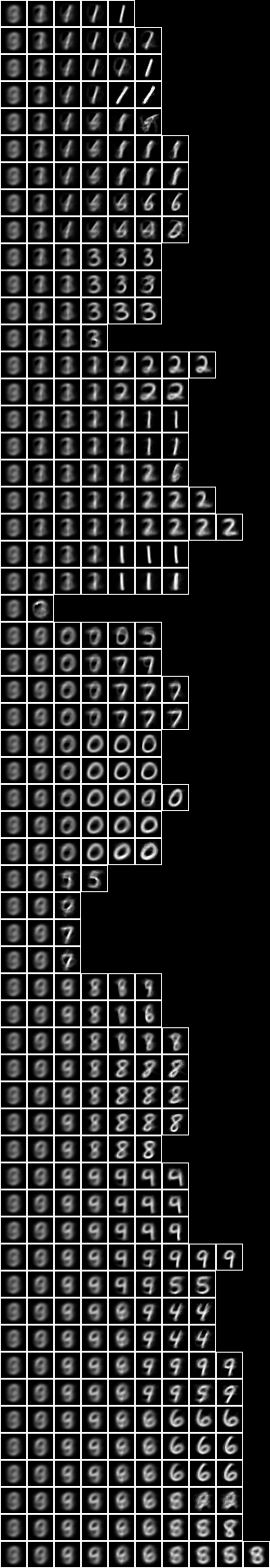Now with the fud underlying superimposed on the averaged slice,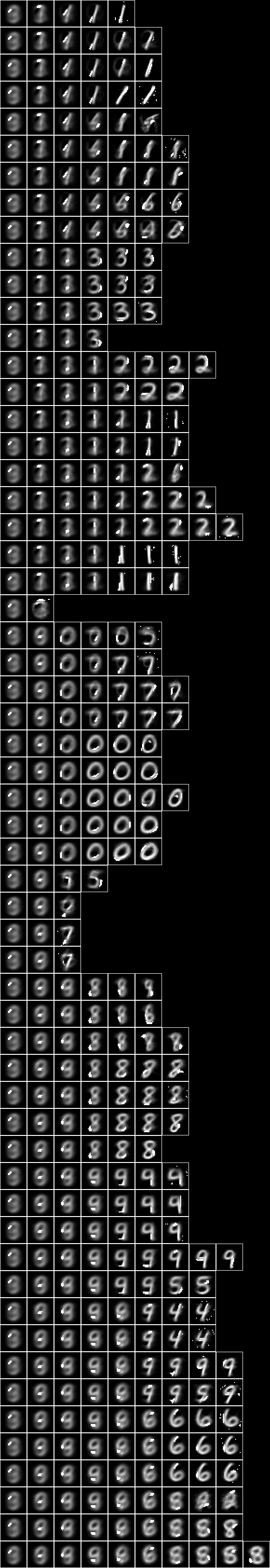### Model 4 - All pixels Similarly, the NIST_model4.json is induced by NIST_engine4.hs. The sample is the entire training set of 60,000 events. The parameters are defined,  let model = "NIST_model4" let (wmax,lmax,xmax,omax,bmax,mmax,umax,pmax,fmax,mult,seed) = (2^11, 8, 2^10, 60, (60*3), 3, 2^8, 1, 127, 1, 5)  This engine runs on a Ubuntu 16.04 Intel(R) Xeon(R) Platinum 8175M CPU @ 2.50GHz using 1673 MB memory in 18875 seconds. The statistics are, model cardinality: 4062 selected train size: 7500 alignment: 143760.91 alignment density: 71292.00  Imaging the fud decomposition,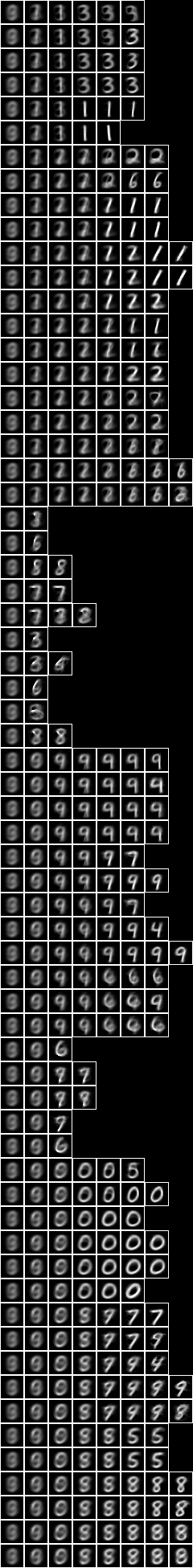Now with the fud underlying superimposed on the averaged slice,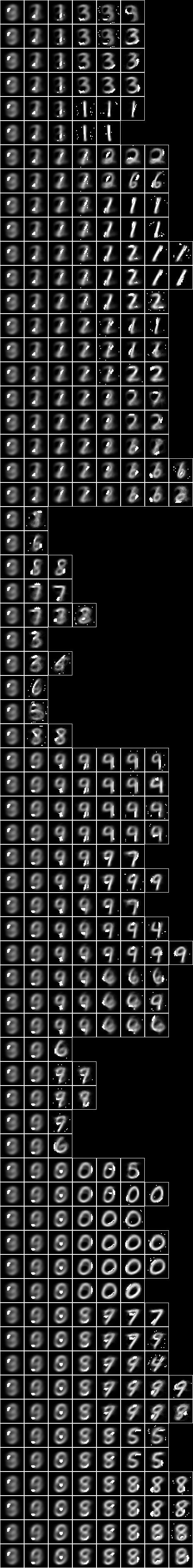### Model 35 - All pixels Similarly, the NIST_model35.json is induced by NIST_engine35.hs. The sample is the entire training set of 60,000 events. The parameters are defined,  let model = "NIST_model35" let (wmax,lmax,xmax,omax,bmax,mmax,umax,pmax,fmax,mult,seed) = (2^11, 8, 2^10, 30, (30*3), 3, 2^8, 1, 127, 1, 5)  This engine runs on a Ubuntu 16.04 Intel(R) Xeon(R) Platinum 8175M CPU @ 2.50GHz using 1410 MB memory in 10759 seconds. The statistics are, model cardinality: 3929 selected train size: 7500 alignment: 129988.66 alignment density: 63698.56  Imaging the fud decomposition,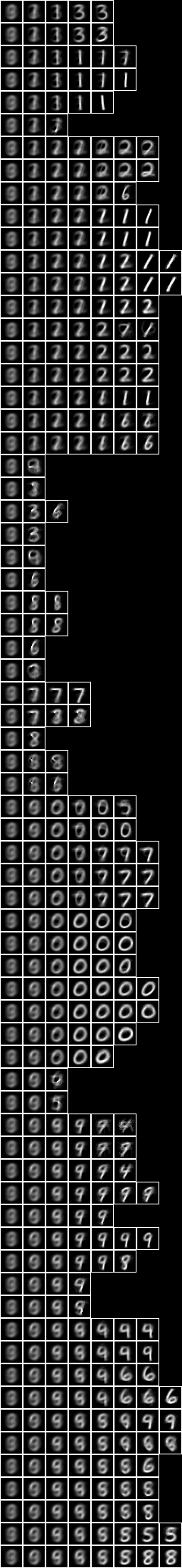Now with the fud underlying superimposed on the averaged slice,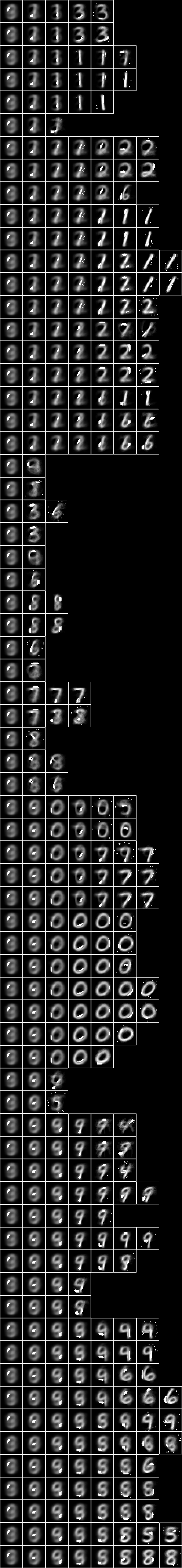### Model 34 - Averaged pixels The pixel variables can be averaged as well as bucketed to reduce the dimension from 28x28 to 9x9. The NIST_model34.json is induced by NIST_engine34.hs. The sample is the entire training set of 60,000 events. The first section loads the sample,  (uu,hr) <- nistTrainBucketedAveragedIO 8 9 0  The parameters are defined,  let model = "NIST_model34" let (wmax,lmax,xmax,omax,bmax,mmax,umax,pmax,fmax,mult,seed) = (2^11, 8, 2^10, 30, (30*3), 3, 2^8, 1, 127, 1, 5)  The engine runs on a Intel(R) Xeon(R) Platinum 8175M CPU @ 2.50GHz using 572 MB memory in 8239 seconds. The statistics are, model cardinality: 4811 selected train size: 7500 alignment: 89445.55 alignment density: 43702.20  Imaging the fud decomposition,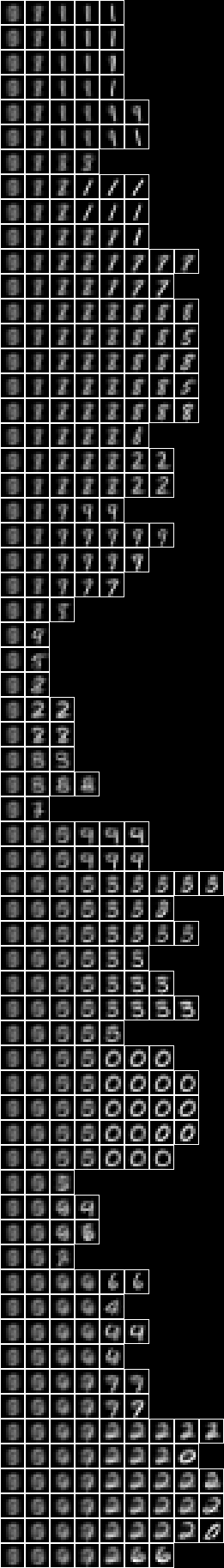Now with the fud underlying superimposed on the averaged slice,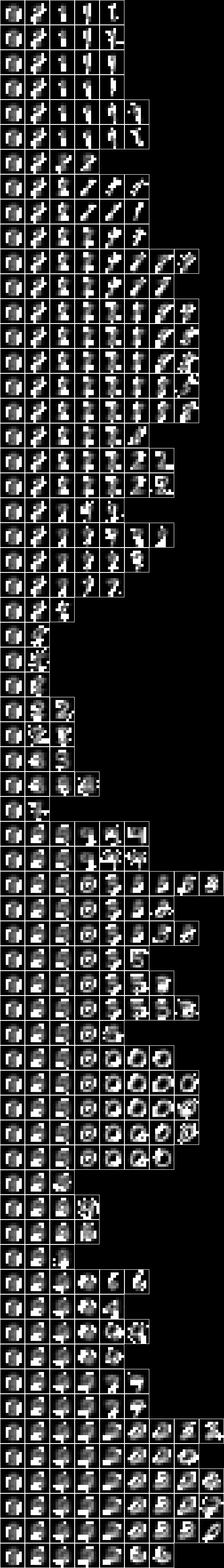### Model 5 - 15-fud square regions of 10x10 pixels Now consider a 15-fud induced model applied to 7500 events of randomly chosen square regions of 10x10 pixels from the training sample. We shall run it in the interpreter, :l NISTDev (uu,hrtr) <- nistTrainBucketedRegionRandomIO 2 10 17 let digit = VarStr "digit" let vv = uvars uu let vvl = sgl digit let vvk = vv minus vvl let hr = hrev [i | i <- [0 .. hrsize hrtr - 1], i mod 8 == 0] hrtr let (wmax,lmax,xmax,omax,bmax,mmax,umax,pmax,fmax,mult,seed) = (2^10, 8, 2^10, 10, (10*3), 3, 2^8, 1, 15, 2, 5) Just (uu1,df) <- decomperIO uu vvk hr wmax lmax xmax omax bmax mmax umax pmax fmax mult seed  This runs in the ghci interpreter on a Ubuntu 16.04 Pentium CPU G2030 @ 3.00GHz in 773 seconds. summation mult seed uu1 df hr (125116.57866462508,62558.28933231254) card dfund df
98

card $fvars$ dfff df
483

BL.writeFile ("NIST_model5.json") $decompFudsPersistentsEncode$ decompFudsPersistent df



Imaging the fud decomposition,

let pp = qqll $treesPaths$ hrmult uu1 df hr

rpln $map (map (hrsize . snd)) pp "[7500,3442,1606,915,677,605]" "[7500,3442,1606,640]" "[7500,3442,1639,1075]" "[7500,3679,1233]" "[7500,3679,2440,741]" "[7500,3679,2440,1673,1011]" bmwrite file$ bmvstack $map (\bm -> bminsert (bmempty ((10*3)+2) (((10*3)+2)*(maximum (map length pp)))) 0 0 bm)$ map (bmhstack . map (\((_,ff),hrs) -> bmborder 1 (bmmax (hrbm 10 3 2 (hrs hrhrred vvk)) 0 0 (hrbm 10 3 2 (qqhr 2 uu vvk (fund ff)))))) pp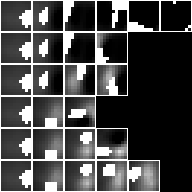Both the averaged slice and the fud underlying are shown in each image to indicate where the attention is during decomposition.

Now showing just the averaged slice,

bmwrite file $bmvstack$ map (\bm -> bminsert (bmempty ((10*3)+2) (((10*3)+2)*(maximum (map length pp)))) 0 0 bm) map (bmhstack . map (\((_,ff),hrs) -> bmborder 1 (hrbm 10 3 2 (hrs hrhrred vvk)))) pp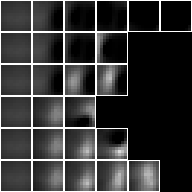### Model 6 - Square regions of 10x10 pixels Now consider a compiled inducer. NIST_model6.json is induced by NIST_engine6.hs. The sample is the entire training set of 60,000 events. The first section loads the sample,  (uu,hr) <- nistTrainBucketedRegionRandomIO 2 10 17  The parameters are defined,  let model = "NIST_model6" let (wmax,lmax,xmax,omax,bmax,mmax,umax,pmax,fmax,mult,seed) = (2^11, 8, 2^10, 30, (30*3), 3, 2^8, 1, 127, 1, 5)  The engine runs on a Intel(R) Xeon(R) Platinum 8175M CPU @ 2.50GHz using 655 MB memory in 9774 seconds. The statistics are, model cardinality: 3659 selected train size: 7500 alignment: 184576.58 alignment density: 91637.01  Imaging the fud decomposition,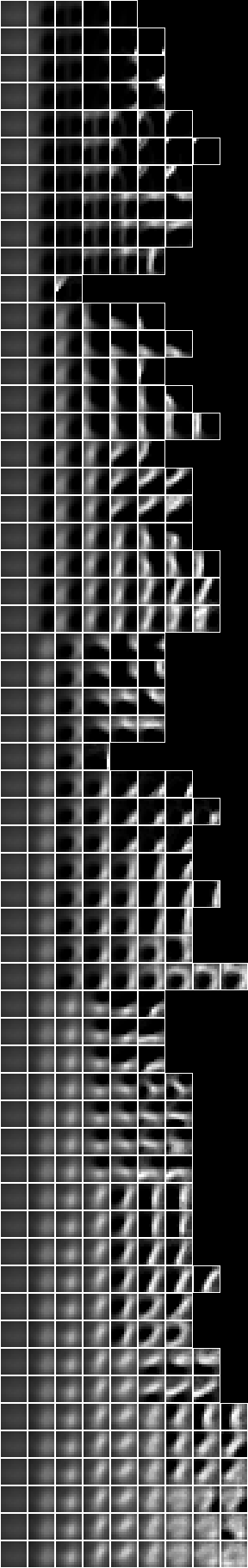Now with the fud underlying superimposed on the averaged slice,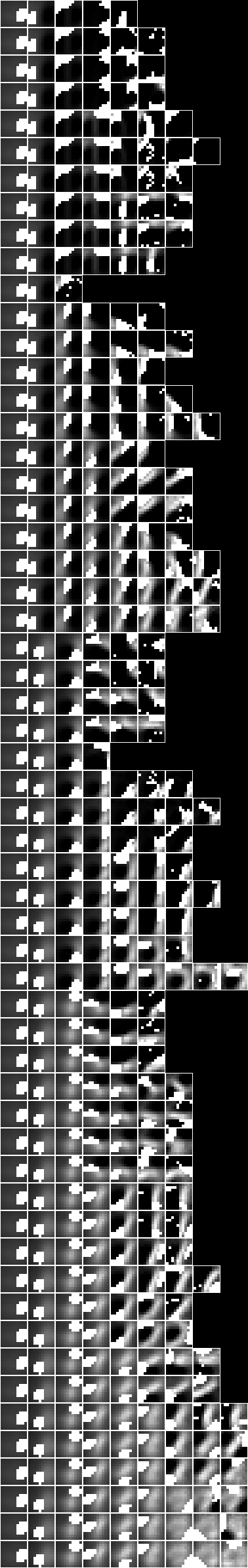### Model 21 - Square regions of 15x15 pixels Now consider a compiled inducer. NIST_model21.json is induced by NIST_engine21.hs. The sample is the entire training set of 60,000 events. The first section loads the sample,  (uu,hr) <- nistTrainBucketedRegionRandomIO 2 15 17  The parameters are defined,  let model = "NIST_model21" let (wmax,lmax,xmax,omax,bmax,mmax,umax,pmax,fmax,mult,seed) = (2^11, 8, 2^10, 30, (30*3), 3, 2^8, 1, 127, 1, 5)  The engine runs on a Intel(R) Xeon(R) Platinum 8175M CPU @ 2.50GHz using 714 MB memory in 10245 seconds. The statistics are, model cardinality: 4086 selected train size: 7500 alignment: 175450.03 alignment density: 87200.26  Imaging the fud decomposition,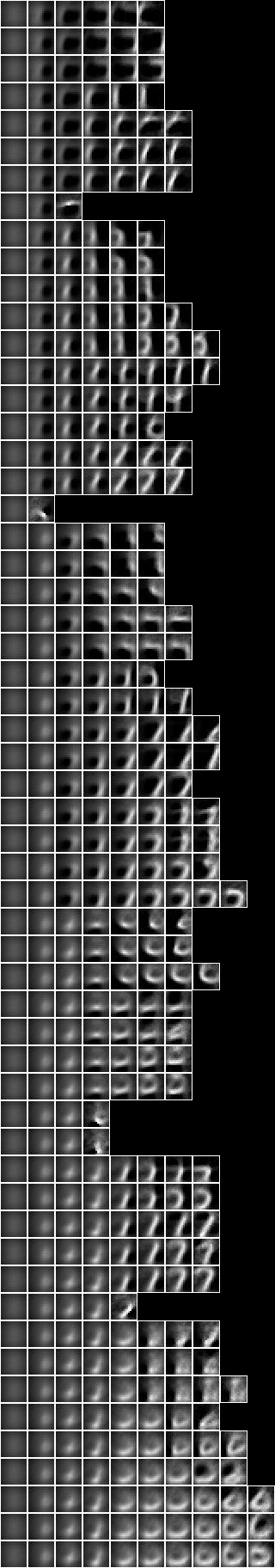Now with the fud underlying superimposed on the averaged slice,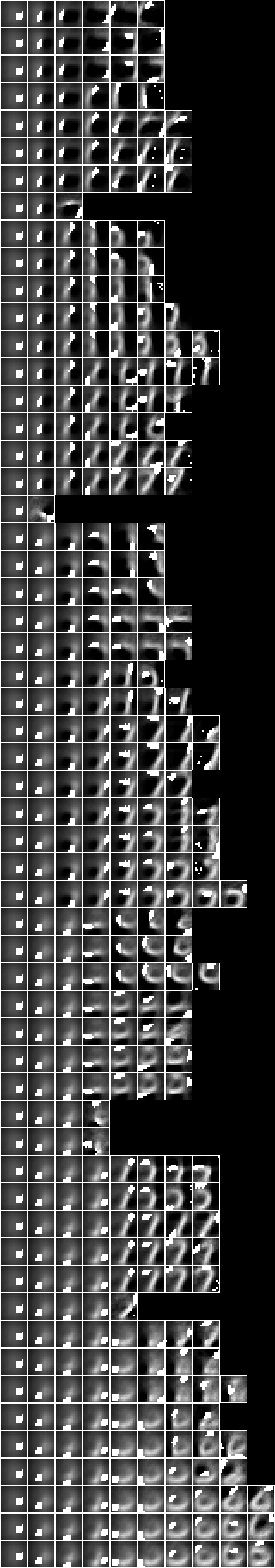### Model 10 - Centred square regions of 11x11 pixels NIST_model10.json is induced by NIST_engine10.hs. The sample is the entire training set of 60,000 events. The first section loads the sample,  (uu,hrtr) <- nistTrainBucketedRegionRandomIO 2 11 17 let u = stringsVariable "<6,6>" let hr = hrtr hrhrsel aahr uu (unit (sgl (llss [(u,ValInt 1)]))) let digit = VarStr "digit" let vv = uvars uu let vvl = sgl digit let vvk = vv minus vvl  Then the parameters are defined,  let model = "NIST_model10" let (wmax,lmax,xmax,omax,bmax,mmax,umax,pmax,fmax,mult,seed) = (2^11, 8, 2^10, 30, (30*3), 3, 2^8, 1, 127, 1, 5)  The engine runs on a Intel(R) Xeon(R) Platinum 8175M CPU @ 2.50GHz using 552 MB memory in 8320 seconds. The statistics are, model cardinality: 3975 selected train size: 7500 alignment: 29422.85 alignment density: 14570.72  Imaging the fud decomposition,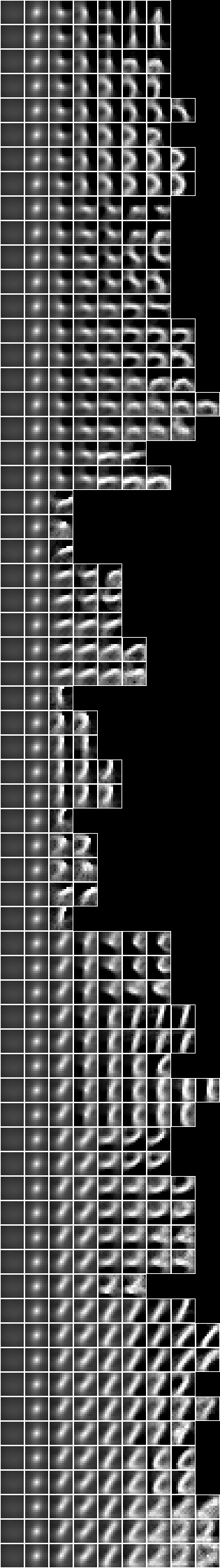Now with the fud underlying superimposed on the averaged slice,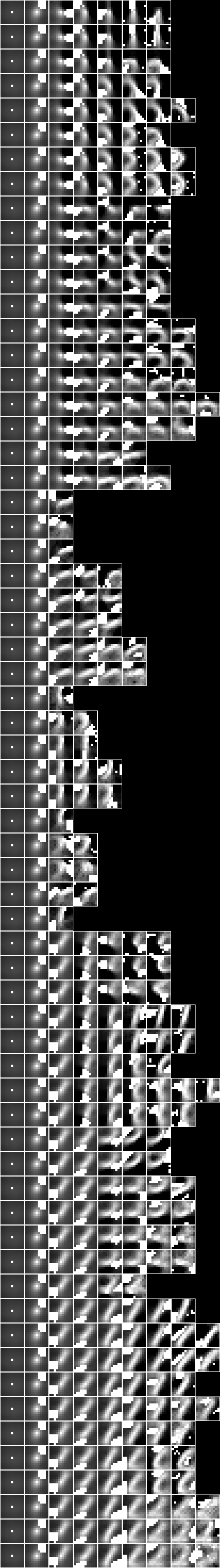### Model 18 - Row and column regions NIST_model18_rows.json and NIST_model18_cols.json are induced by NIST_engine18.hs. The sample is the entire training set of 60,000 events. The first section loads the sample. For columns,  (uu,hr) <- nistTrainBucketedRectangleRandomIO 2 28 1 17  or for rows,  (uu,hr) <- nistTrainBucketedRectangleRandomIO 2 1 28 17 let digit = VarStr "digit" let vv = uvars uu let vvl = sgl digit let vvk = vv minus vvl  Then the parameters are defined,  let model = "NIST_model18_cols"  or for rows,  let model = "NIST_model18_rows" let (wmax,lmax,xmax,omax,bmax,mmax,umax,pmax,fmax,mult,seed) = (2^11, 8, 2^10, 30, (30*3), 3, 2^8, 1, 127, 1, 5)  The engine runs on a Intel(R) Xeon(R) Platinum 8175M CPU @ 2.50GHz using 737 MB memory in 13967 seconds. Then the decomper is run,  Just (uu1,df1) <- decomperIO uu vvk hr wmax lmax xmax omax bmax mmax umax pmax fmax mult seed  Then the models are written to NIST_model18_rows.json and NIST_model18_cols.json. ### Model 24 - Two level over 10x10 regions Now consider 5x5 copies of the Model 6 - Square regions of 10x10 pixels over the whole query substrate. NIST_model24.json is induced by NIST_engine24.hs. The sample is the entire training set of 60,000 events. The first section loads the underlying sample,  (uu,hr) <- nistTrainBucketedRegionRandomIO 2 10 17  Then the underlying decomposition is loaded, reduced, converted to a nullable fud, and reframed at level 1,  s <- BL.readFile "./NIST_model6.json" let df1 = fromJust persistentsDecompFud $fromJust$ (Data.Aeson.decode s :: Maybe DecompFudPersistent)

let uu1 = uu uunion (fsys (dfff df1))

let ff1 = fframe (refr1 1) $dfnul uu1 (dfred uu1 df1 hr) 1  where dfred = systemsDecompFudsHistoryRepasDecompFudReduced dfnul uu df g = fromJust$ systemsDecompFudsNullablePracticable uu df g

refr1 k (VarPair (VarPair (VarInt f, l), VarInt i)) = VarPair (VarPair (VarPair (VarPair (VarInt k, VarInt f), VarInt 0), l), VarInt i)
refr1 k (VarPair (VarPair (VarPair (VarInt f, g), l), VarInt i)) = VarPair (VarPair (VarPair (VarPair (VarInt k, VarInt f), g), l), VarInt i)
refr1 _ v = v


    (uu,hr) <- nistTrainBucketedIO 2

let digit = VarStr "digit"
let vv = uvars uu
let vvl = sgl digit
let vvk = vv minus vvl


Then the underlying model is copied and reframed at level 2,

    let gg1 = foldl funion fudEmpty [fframe (refr2 x y) ff1 | x <- [2,6,10,14,18], y <- [2,6,10,14,18]]

let uu1 = uu uunion (fsys gg1)


where

refr2 x y (VarPair (VarInt i, VarInt j)) = VarPair (VarInt ((x-1)+i), VarInt ((y-1)+j))
refr2 x y v = VarPair (v, VarStr ("(" ++ show x ++ ";" ++ show y ++ ")"))


Then the parameters are defined,

    let model = "NIST_model24"
let (wmax,lmax,xmax,omax,bmax,mmax,umax,pmax,fmax,mult,seed) = (2^11, 8, 2^10, 30, (30*3), 3, 2^8, 1, 127, 1, 5)


Then the decomper is run,

    Just (uu2,df2) <- decomperIO uu1 gg1 hr wmax lmax xmax omax bmax mmax umax pmax fmax mult seed
...
where
...
decomperIO uu ff aa wmax lmax xmax omax bmax mmax umax pmax fmax mult seed =
parametersSystemsHistoryRepasDecomperLevelMaxRollByMExcludedSelfHighestFmaxIORepa wmax lmax xmax omax bmax mmax umax pmax fmax mult seed uu (Tree Map.singleton (wmax,Set.empty,ff) emptyTree) aa  The engine runs on a Intel(R) Xeon(R) Platinum 8175M CPU @ 2.50GHz using 28791 MB memory in 28540 seconds. The statistics are, model cardinality: 6158 selected train size: 7500 alignment: 197806.78 alignment density: 94133.43  Imaging the fud decomposition,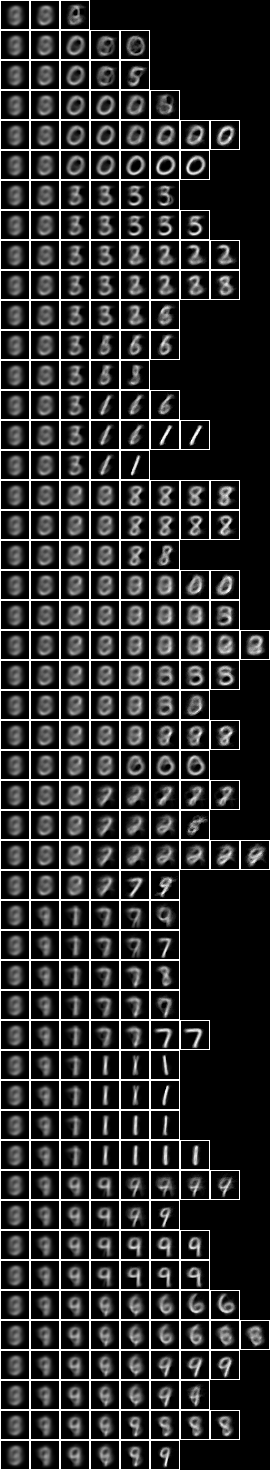Now with the fud underlying superimposed on the averaged slice,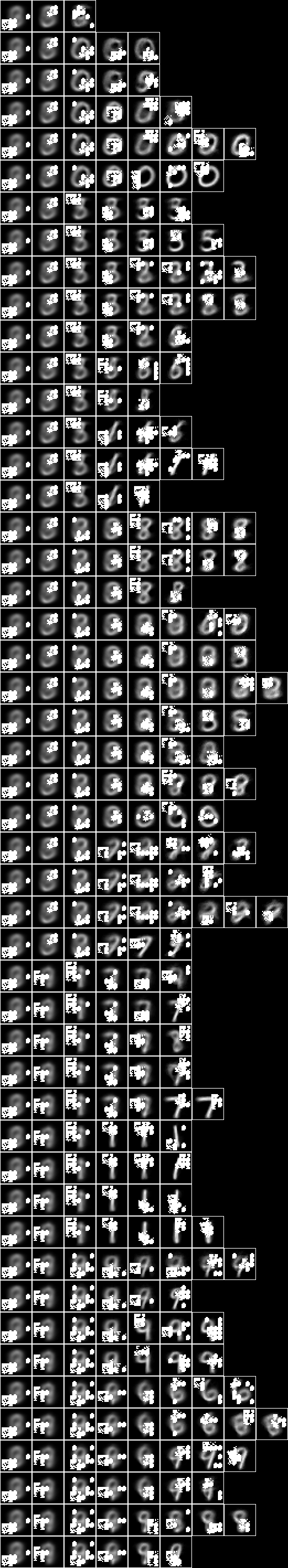### Model 25 - Two level over 15x15 regions Now consider 5x5 copies of the Model 21 - Square regions of 15x15 pixels over the whole query substrate. NIST_model25.json is induced by NIST_engine25.hs. The sample is the entire training set of 60,000 events. The engine is very similar to Model 24 - Two level over 10x10 regions. The first section loads the underlying sample,  (uu,hr) <- nistTrainBucketedRegionRandomIO 2 15 17  Then the underlying decomposition is loaded, reduced, converted to a nullable fud, and reframed at level 1,  s <- BL.readFile "./NIST_model21.json" let df1 = fromJust persistentsDecompFud $fromJust$ (Data.Aeson.decode s :: Maybe DecompFudPersistent)

let uu1 = uu uunion (fsys (dfff df1))

let ff1 = fframe (refr1 1) $dfnul uu1 (dfred uu1 df1 hr) 1  where dfred = systemsDecompFudsHistoryRepasDecompFudReduced dfnul uu df g = fromJust$ systemsDecompFudsNullablePracticable uu df g

refr1 k (VarPair (VarPair (VarInt f, l), VarInt i)) = VarPair (VarPair (VarPair (VarPair (VarInt k, VarInt f), VarInt 0), l), VarInt i)
refr1 k (VarPair (VarPair (VarPair (VarInt f, g), l), VarInt i)) = VarPair (VarPair (VarPair (VarPair (VarInt k, VarInt f), g), l), VarInt i)
refr1 _ v = v


    (uu,hr) <- nistTrainBucketedIO 2

let digit = VarStr "digit"
let vv = uvars uu
let vvl = sgl digit
let vvk = vv minus vvl


Then the underlying model is copied and reframed at level 2,

    let gg1 = foldl funion fudEmpty [fframe (refr2 x y) ff1 | x <- [1,4,7,10,13], y <- [1,4,7,10,13]]

let uu1 = uu uunion (fsys gg1)


where

refr2 x y (VarPair (VarInt i, VarInt j)) = VarPair (VarInt ((x-1)+i), VarInt ((y-1)+j))
refr2 x y v = VarPair (v, VarStr ("(" ++ show x ++ ";" ++ show y ++ ")"))


Then the parameters are defined,

    let model = "NIST_model25"
let (wmax,lmax,xmax,omax,bmax,mmax,umax,pmax,fmax,mult,seed) = (2^11, 8, 2^10, 30, (30*3), 3, 2^8, 1, 127, 1, 5)


Then the decomper is run,

    Just (uu2,df2) <- decomperIO uu1 gg1 hr wmax lmax xmax omax bmax mmax umax pmax fmax mult seed
...
where
...
decomperIO uu ff aa wmax lmax xmax omax bmax mmax umax pmax fmax mult seed =
parametersSystemsHistoryRepasDecomperLevelMaxRollByMExcludedSelfHighestFmaxIORepa wmax lmax xmax omax bmax mmax umax pmax fmax mult seed uu (Tree Map.singleton (wmax,Set.empty,ff) emptyTree) aa  The engine runs on a Intel(R) Xeon(R) Platinum 8175M CPU @ 2.50GHz using 29036 MB memory in 30648 seconds. The statistics are, model cardinality: 5194 selected train size: 7500 alignment: 197744.69 alignment density: 96419.64  Imaging the fud decomposition,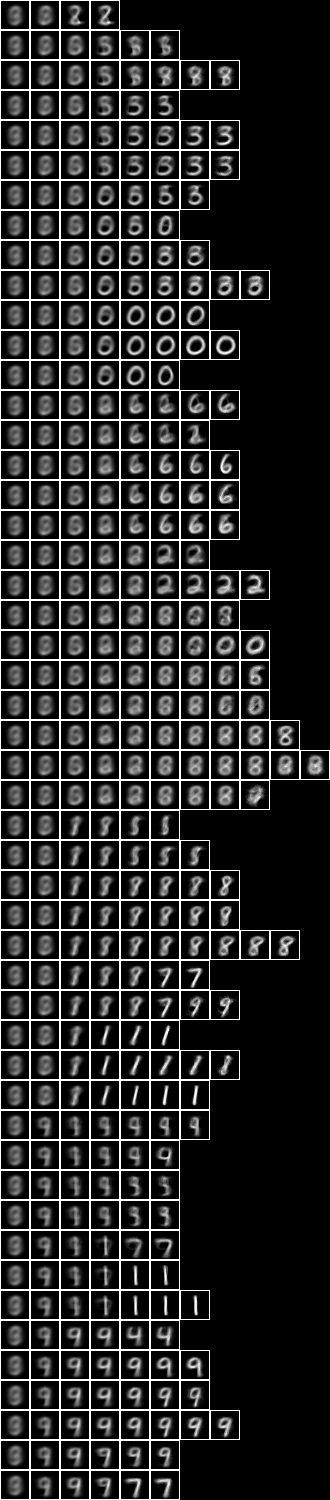Now with the fud underlying superimposed on the averaged slice,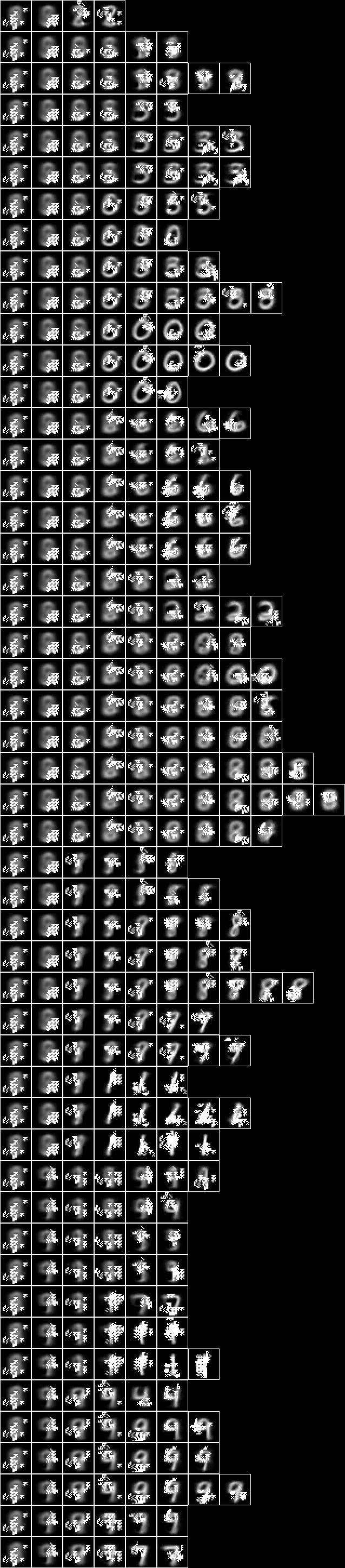### Model 26 - Two level over centred square regions of 11x11 pixels Now consider 5x5 copies of the Model 10 - Centred square regions of 11x11 pixels over the whole query substrate. NIST_model26.json is induced by NIST_engine26.hs. The sample is the entire training set of 60,000 events. The engine is very similar to Model 24 - Two level over 10x10 regions and Model 25 - Two level over 15x15 regions. The first section loads the underlying sample,  (uu,hr) <- nistTrainBucketedRegionRandomIO 2 11 17  Then the underlying decomposition is loaded, reduced, converted to a nullable fud, and reframed at level 1,  s <- BL.readFile "./NIST_model10.json" let df1 = fromJust persistentsDecompFud $fromJust$ (Data.Aeson.decode s :: Maybe DecompFudPersistent)

let uu1 = uu uunion (fsys (dfff df1))

let ff1 = fframe (refr1 1) $dfnul uu1 (dfred uu1 df1 hr) 1  where dfred = systemsDecompFudsHistoryRepasDecompFudReduced dfnul uu df g = fromJust$ systemsDecompFudsNullablePracticable uu df g

refr1 k (VarPair (VarPair (VarInt f, l), VarInt i)) = VarPair (VarPair (VarPair (VarPair (VarInt k, VarInt f), VarInt 0), l), VarInt i)
refr1 k (VarPair (VarPair (VarPair (VarInt f, g), l), VarInt i)) = VarPair (VarPair (VarPair (VarPair (VarInt k, VarInt f), g), l), VarInt i)
refr1 _ v = v


    (uu,hr) <- nistTrainBucketedIO 2

let digit = VarStr "digit"
let vv = uvars uu
let vvl = sgl digit
let vvk = vv minus vvl


Then the underlying model is copied and reframed at level 2,

    let gg1 = foldl funion fudEmpty [fframe (refr2 x y) ff1 | x <- [2,6,10,14,18], y <- [2,6,10,14,18]]

let uu1 = uu uunion (fsys gg1)


where

refr2 x y (VarPair (VarInt i, VarInt j)) = VarPair (VarInt ((x-1)+i), VarInt ((y-1)+j))
refr2 x y v = VarPair (v, VarStr ("(" ++ show x ++ ";" ++ show y ++ ")"))


Then the parameters are defined,

    let model = "NIST_model26"
let (wmax,lmax,xmax,omax,bmax,mmax,umax,pmax,fmax,mult,seed) = (2^11, 8, 2^10, 30, (30*3), 3, 2^8, 1, 127, 1, 5)


Then the decomper is run,

    Just (uu2,df2) <- decomperIO uu1 gg1 hr wmax lmax xmax omax bmax mmax umax pmax fmax mult seed
...
where
...
decomperIO uu ff aa wmax lmax xmax omax bmax mmax umax pmax fmax mult seed =
parametersSystemsHistoryRepasDecomperLevelMaxRollByMExcludedSelfHighestFmaxIORepa wmax lmax xmax omax bmax mmax umax pmax fmax mult seed uu (Tree Map.singleton (wmax,Set.empty,ff) emptyTree) aa  The engine runs on a Intel(R) Xeon(R) Platinum 8175M CPU @ 2.50GHz using 24516 MB memory in 28273 seconds. The statistics are, model cardinality: 7701 selected train size: 7500 alignment: 158648.92 alignment density: 78295.49  Imaging the fud decomposition,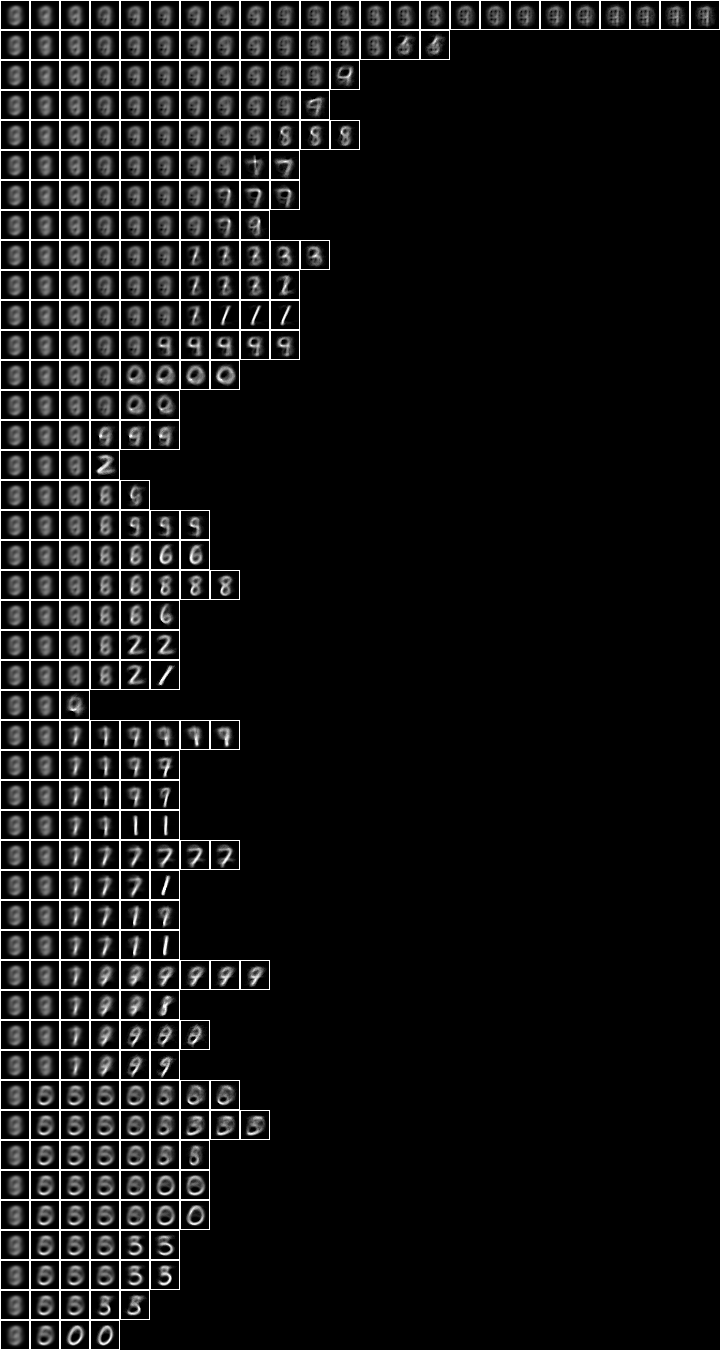Now with the fud underlying superimposed on the averaged slice,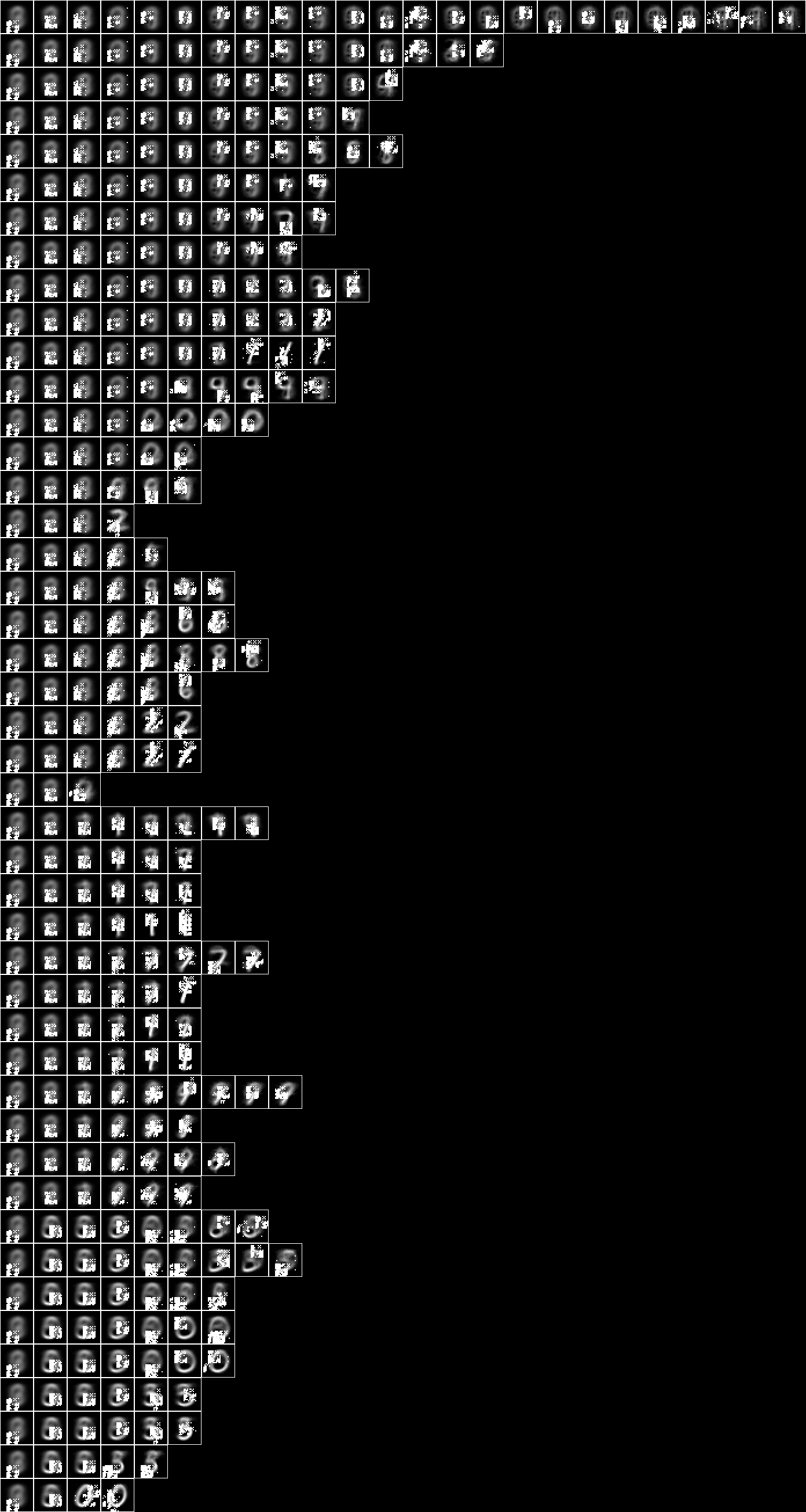### NIST test Some of the models above are very large, so the label tests are best run compiled. The NIST_test.hs is compiled and executed as follows, cd ../Alignment rm *.o *.hi cd ../AlignmentRepa rm *.o *.hi gcc -fPIC -c AlignmentForeign.c -o AlignmentForeign.o -O3 cd ../NIST rm *.o *.hi ghc -i../Alignment -i../AlignmentRepa ../AlignmentRepa/AlignmentForeign.o NIST_test.hs -o NIST_test.exe -rtsopts -O2 ./NIST_test.exe NIST_model2 +RTS -s model: NIST_model2 selected train size: 7500 model cardinality: 542 nullable fud cardinality: 711 nullable fud derived cardinality: 143 nullable fud underlying cardinality: 147 ff label ent: 1.3524518741683345 test size: 1000 effective size: 986 % 1 matches: 463  This runs on model 2 on a Ubuntu 16.04 Pentium CPU G2030 @ 3.00GHz in 470 MB memory in 86 seconds. The first section loads the sample and the model,  [model] <- getArgs (uu,hrtr) <- nistTrainBucketedIO 2 let hr = hrev [i | i <- [0.. hrsize hrtr - 1], i mod 8 == 0] hrtr let digit = VarStr "digit" let vv = uvars uu let vvl = sgl digit let vvk = vv minus vvl s <- BL.readFile (model ++ ".json") let df1 = fromJust persistentsDecompFud $fromJust$ (Data.Aeson.decode s :: Maybe DecompFudPersistent)


Then the fud decomposition fud is created,

    let uu1 = uu uunion (fsys (dfff df1))
let ff1 = fromJust $systemsDecompFudsNullablePracticable uu1 df1 9  Then the label entropy is calculated,  let uu1 = uu uunion (fsys ff1) let hr1 = hrfmul uu1 ff1 hr printf "ff label ent: %.16f\n"$ hrlent uu1 hr1 (fder ff1) vvl


Lastly, the test history is loaded and the query effectiveness and query accuracy is calculated,

    (uu,hrte) <- nistTestBucketedIO 2
let hrq = hrev [i | i <- [0 .. hrsize hrte - 1], i mod 10 == 0] hrte
let hrq1 = hrfmul uu1 ff1 hrq
printf "effective size: %s\n" $rp$ size $hhaa (hrhh uu1 (hrq1 hrhrred (fder ff1))) mul eff (hhaa (hrhh uu1 (hr1 hrhrred (fder ff1)))) printf "matches: %d\n"$ length [rr | let hhq = hrhh uu1 (hrq1 hrhrred (fder ff1 union vvl)), let aa = hhaa (hrhh uu1 (hr1 hrhrred (fder ff1 union vvl))), (_,ss) <- hhll hhq, let qq = single ss 1, let rr = aa mul (qq red fder ff1) red vvl, size rr > 0, size (amax rr mul (qq red vvl)) > 0]


### NIST conditional

Given an induced model, this engine finds a semi-supervised submodel that predicts the label variables, $V_{\mathrm{l}}$, or digit, by optimising conditional entropy. The NIST_engine_cond.hs is compiled as follows,

cd ../Alignment
rm *.o *.hi
cd ../AlignmentRepa
rm *.o *.hi
gcc -fPIC -c AlignmentForeign.c -o AlignmentForeign.o -O3
cd ../NIST
rm *.o *.hi
ghc -i../Alignment -i../AlignmentRepa ../AlignmentRepa/AlignmentForeign.o NIST_engine_cond.hs -o NIST_engine_cond.exe -rtsopts -O2


The first section loads the parameters, sample and the model,

    [valency_s,modelin,kmax_s,omax_s,fmax_s,model] <- getArgs
(uu,hr) <- nistTrainBucketedIO valency
let digit = VarStr "digit"
let vv = uvars uu
let vvl = sgl digit
let vvk = vv minus vvl
df1 <- dfIO  (modelin ++ ".json")


Then the fud decomposition fud is created,

    let uu1 = uu uunion (fsys (dfff df1))
let ff1 = fframe (refr1 3) $dfnul uu1 df1 3  Then the model is applied,  let uu1 = uu uunion (fsys ff1) let hr1 = hrfmul uu1 ff1 hr  Then the conditional entropy fud decomper is run,  let (uu2,df2) = decompercondrr vvl uu1 hr1 kmax omax fmax let df2' = zzdf$ funcsTreesMap (\(ss,ff) -> (ss, (ff funion ff1) fdep fder ff)) $dfzz df2  where decompercondrr vvl uu aa kmax omax fmax = fromJust$ parametersSystemsHistoryRepasDecomperConditionalFmaxRepa kmax omax fmax uu vvl aa


Then the model is written to NIST_model36.json,

    BL.writeFile (model ++ ".json") $decompFudsPersistentsEncode$ decompFudsPersistent df2'


Finally the images are written out,

    let pp = qqll $treesPaths$ hrmult uu2 df2' hr'
bmwrite (model ++ ".bmp") $bmvstack$ map (\bm -> bminsert (bmempty (28+2) ((28+2)*(maximum (map length pp)))) 0 0 bm) $map (bmhstack . map (\(_,hrs) -> bmborder 1 (hrbm 28 1 2 (hrs hrhrred vvk))))$ pp
bmwrite (model ++ "_1.bmp") $bmvstack$ map (\bm -> bminsert (bmempty ((28*1)+2) (((28*1)+2)*(maximum (map length pp)))) 0 0 bm) $map (bmhstack . map (\((_,ff),hrs) -> bmborder 1 (bmmax (hrbm 28 1 2 (hrs hrhrred vvk)) 0 0 (hrbm 28 1 2 (qqhr 2 uu vvk (fund ff))))))$ pp
bmwrite (model ++ "_2.bmp") $bmvstack$ map (\bm -> bminsert (bmempty ((28*2)+2) (((28*2)+2)*(maximum (map length pp)))) 0 0 bm) $map (bmhstack . map (\((_,ff),hrs) -> bmborder 1 (bmmax (hrbm 28 2 2 (hrs hrhrred vvk)) 0 0 (hrbm 28 2 2 (qqhr 2 uu vvk (fund ff))))))$ pp


### Model 36 - Conditional level over 15-fud

Now run the conditional entropy fud decomper to create Model 36, the conditional level over 15-fud induced model of all pixels, Model 2,

./NIST_engine_cond.exe 2 NIST_model2 1 5 15 NIST_model36 +RTS -s >NIST_engine36.log 2>&1


This runs on on a Ubuntu 16.04 Pentium CPU G2030 @ 3.00GHz in 1754 MB memory in 180 seconds.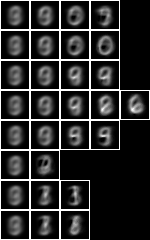Now with the fud underlying superimposed on the averaged slice,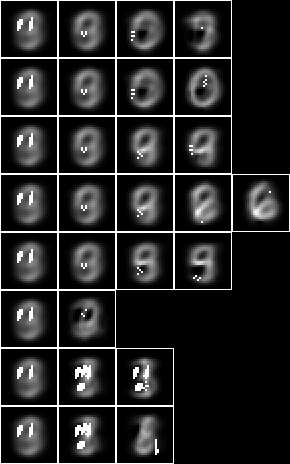If we run NIST test on the new model,

./NIST_test.exe NIST_model36 +RTS -s >NIST_test_model36.log 2>&1


we obtain the following statistics,

model: NIST_model36
selected train size: 7500
model cardinality: 206
nullable fud cardinality: 246
nullable fud derived cardinality: 15
nullable fud underlying cardinality: 87
ff label ent: 1.3402902066958342
test size: 1000
effective size: 1000 % 1
matches: 534


### Model 37 - Conditional level over all pixels

Now let us repeat the analysis of Model 36, but for the conditional level over 127-fud induced model of all pixels, Model 35. Model 37 -

./NIST_engine_cond.exe 2 NIST_model35 1 5 127 NIST_model37 +RTS -s >NIST_engine37.log 2>&1


This runs on on a Ubuntu 16.04 AMD EPYC 7571 cpu @ 2199.522 MHz in 9380 MB memory in 4705 seconds.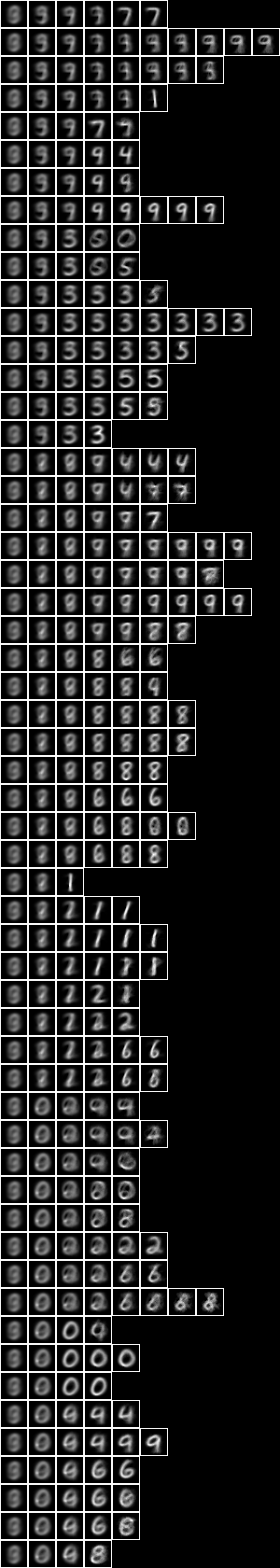Now with the fud underlying superimposed on the averaged slice,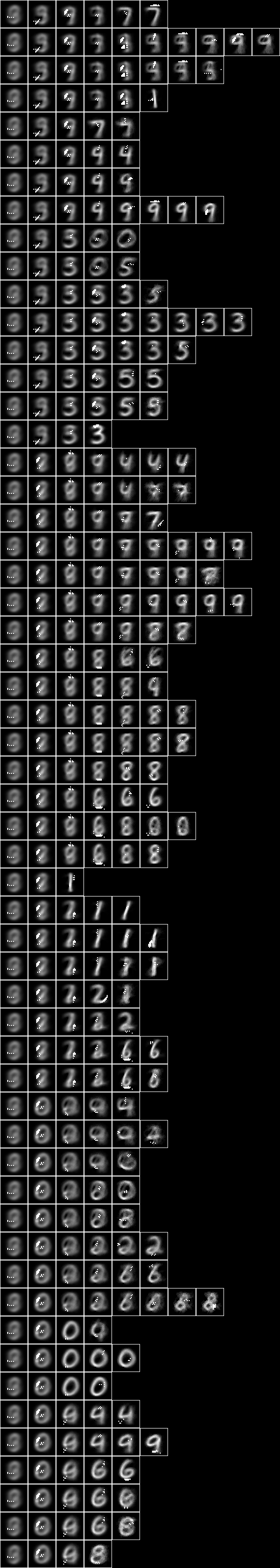If we run NIST test on the new model,

./NIST_test.exe NIST_model37 +RTS -s >NIST_test_model37.log 2>&1


we obtain the following statistics,

model: NIST_model37
selected train size: 7500
model cardinality: 650
nullable fud cardinality: 1026
nullable fud derived cardinality: 127
nullable fud underlying cardinality: 255
ff label ent: 0.5951985228651324
test size: 1000
effective size: 999 % 1
matches: 814


Repeating the same, but with 2-tuple conditional,

./NIST_engine_cond.exe 2 NIST_model35 2 5 127 NIST_model39 +RTS -s >NIST_engine39.log 2>&1


This runs on on a Ubuntu 16.04 AMD EPYC 7571 cpu @ 2199.522 MHz in 7167 MB memory in 24283 seconds.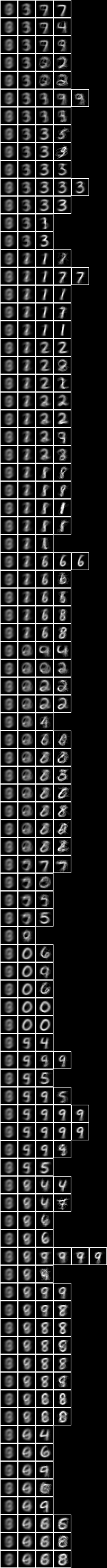Now with the fud underlying superimposed on the averaged slice,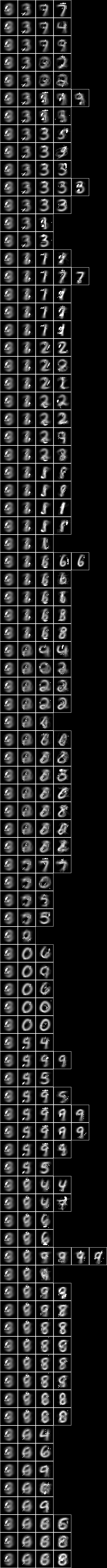If we run NIST test on the new model,

./NIST_test.exe NIST_model39 +RTS -s >NIST_test_model39.log 2>&1


we obtain the following statistics,

model: NIST_model39
selected train size: 7500
model cardinality: 883
nullable fud cardinality: 1253
nullable fud derived cardinality: 127
nullable fud underlying cardinality: 322
ff label ent: 0.3877749488432780
test size: 1000
effective size: 985 % 1
matches: 824


### Model 38 - Conditional level over averaged pixels

Now let us repeat the analysis for the conditional level over 127-fud induced model of averaged pixels, Model 34.

The NIST_engine_cond_averaged.hs is compiled as follows,

cd ../Alignment
rm *.o *.hi
cd ../AlignmentRepa
rm *.o *.hi
gcc -fPIC -c AlignmentForeign.c -o AlignmentForeign.o -O3
cd ../NIST
rm *.o *.hi
ghc -i../Alignment -i../AlignmentRepa ../AlignmentRepa/AlignmentForeign.o NIST_engine_cond_averaged.hs -o NIST_engine_cond_averaged.exe -rtsopts -O2


The first section loads the parameters, sample and the model,

    [valency_s,breadth_s,offset_s,modelin,kmax_s,omax_s,fmax_s,model] <- getArgs
(uu,hr) <- nistTrainBucketedAveragedIO valency breadth offset
let digit = VarStr "digit"
let vv = uvars uu
let vvl = sgl digit
let vvk = vv minus vvl
df1 <- dfIO  (modelin ++ ".json")


The remainder is as for Model 36.

Now run Model 38 -

./NIST_engine_cond_averaged.exe 8 9 0 NIST_model34 1 5 127 NIST_model38 +RTS -s >NIST_engine38.log 2>&1


This runs on on a Ubuntu 16.04 AMD EPYC 7571 cpu @ 2199.522 MHz in 9068 MB memory in 5094 seconds.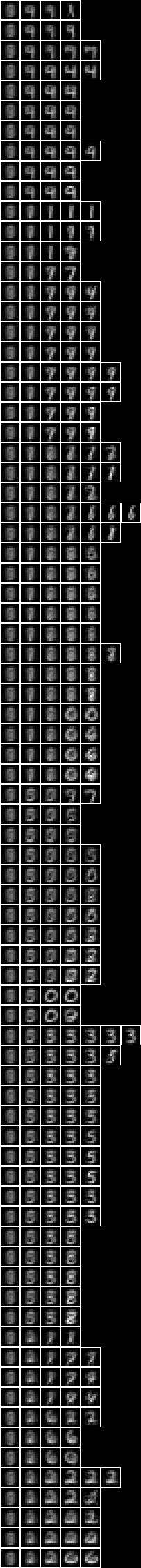Now with the fud underlying superimposed on the averaged slice,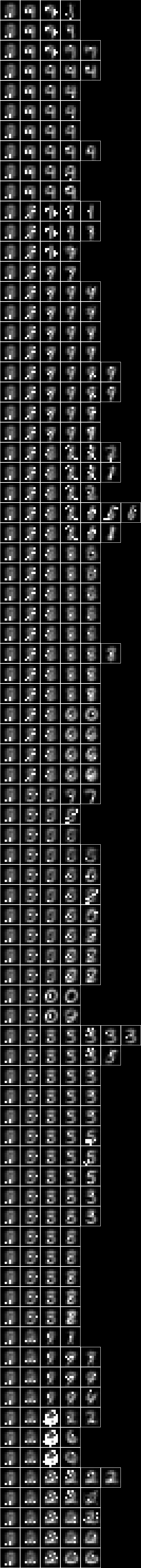### NIST test averaged

We must modify NIST test to allow for an averaged substrate. The NIST_test_averaged.hs is compiled and executed as follows,

cd ../Alignment
rm *.o *.hi
cd ../AlignmentRepa
rm *.o *.hi
gcc -fPIC -c AlignmentForeign.c -o AlignmentForeign.o -O3
cd ../NIST
rm *.o *.hi
ghc -i../Alignment -i../AlignmentRepa ../AlignmentRepa/AlignmentForeign.o NIST_test_averaged.hs -o NIST_test_averaged.exe -rtsopts -O2

./NIST_test_averaged.exe 8 9 0 NIST_model38 +RTS -s >NIST_test_model38.log 2>&1


we obtain the following statistics,

model: NIST_model38
selected train size: 7500
model cardinality: 419
nullable fud cardinality: 794
nullable fud derived cardinality: 127
nullable fud underlying cardinality: 46
ff label ent: 0.6267873188842659
test size: 1000
effective size: 990 % 1
matches: 701


### NIST conditional over square regions

We can modify NIST conditional to copy an array of region models. The NIST_engine_cond_regions.hs is compiled and executed as follows,

cd ../Alignment
rm *.o *.hi
cd ../AlignmentRepa
rm *.o *.hi
gcc -fPIC -c AlignmentForeign.c -o AlignmentForeign.o -O3
cd ../NIST
rm *.o *.hi
ghc -i../Alignment -i../AlignmentRepa ../AlignmentRepa/AlignmentForeign.o NIST_engine_cond_regions.hs -o NIST_engine_cond_regions.exe -rtsopts -O2


The first section loads the parameters, regional sample and the regional model,

    [valency_s,breadth_s,seed_s,ufmax_s,locations_s,modelin,kmax_s,omax_s,fmax_s,model] <- getArgs
let [ufmax,kmax,omax,fmax] = map read [ufmax_s,kmax_s,omax_s,fmax_s] :: [Integer]
let locations = map read $words locations_s (uu,hr) <- nistTrainBucketedRegionRandomIO valency breadth seed df1 <- dfIO (modelin ++ ".json")  Then the fud decomposition fud is created,  let uu1 = uu uunion (fsys (dfff df1)) let ff1 = fframe (refr1 3)$ dfnul uu1 (df1 dflt ufmax) 3


    (uu,hr) <- nistTrainBucketedIO valency
let digit = VarStr "digit"
let vv = uvars uu
let vvl = sgl digit
let vvk = vv minus vvl


Then the model is copied,

    let gg1 = foldl funion fudEmpty [fframe (refr2 x y) ff1 | x <- locations, y <- locations]


Then the model is applied,

    let uu1 = uu uunion (fsys gg1)
let hr1 = hrfmul uu1 gg1 hr


Then the conditional entropy fud decomper is run,

    let (uu2,df2) = decompercondrr vvl uu1 hr1 kmax omax fmax
let df2' = zzdf $funcsTreesMap (\(ss,ff) -> (ss, (ff funion gg1) fdep fder ff))$ dfzz df2


where

decompercondrr vvl uu aa kmax omax fmax = fromJust \$ parametersSystemsHistoryRepasDecomperConditionalFmaxRepa kmax omax fmax uu vvl aa


The remainder of the engine is as for NIST conditional.

### Model 43 - Conditional level over square regions of 15x15 pixels

Now run the regional conditional entropy fud decomper to create Model 43, the conditional level over a 7x7 array of 127-fud induced models of square regions of 15x15 pixels, Model 21,

./NIST_engine_cond_regions.exe 2 15 17 31 "1 3 5 7 9 11 13" NIST_model21 1 5 127 NIST_model43 +RTS -s >NIST_engine43.log 2>&1


This runs on on a Ubuntu 16.04 AMD EPYC 7571 cpu @ 2199.522 MHz in 35928 MB memory in 24283 seconds.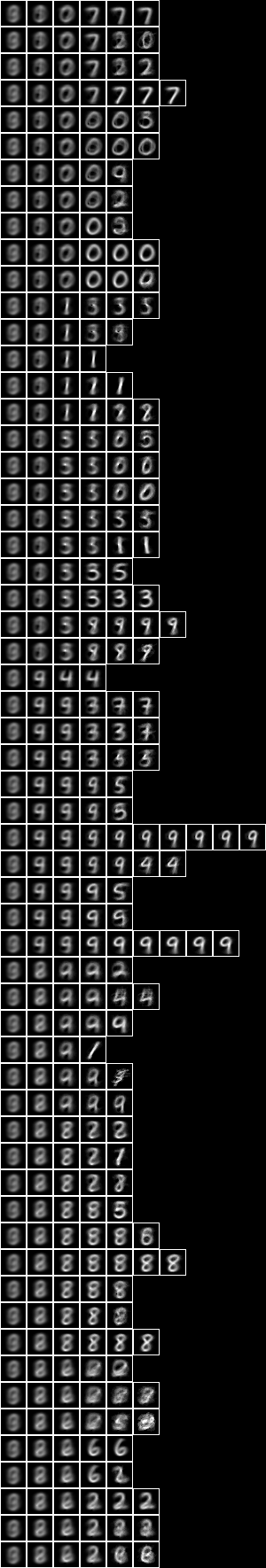Now with the fud underlying superimposed on the averaged slice,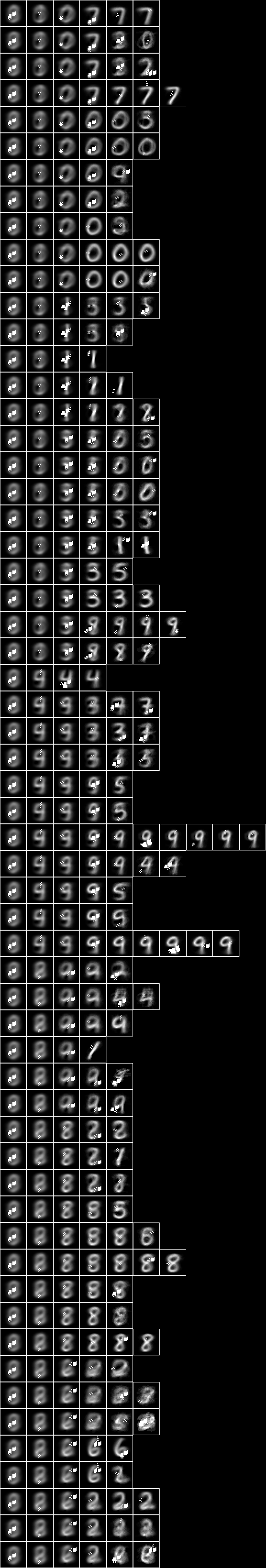If we run NIST test on the new model,

./NIST_test.exe NIST_model43 +RTS -s >NIST_test_model43.log 2>&1


we obtain the following statistics,

model: NIST_model43
selected train size: 7500
model cardinality: 2034
nullable fud cardinality: 2410
nullable fud derived cardinality: 127
nullable fud underlying cardinality: 361
ff label ent: 0.5642948913994976
test size: 1000
effective size: 997 % 1
matches: 808


### Model 40 - Conditional level over two level over 10x10 regions

Now run the conditional entropy fud decomper over the 2-level induced model Model 24 to create Model 40,

./NIST_engine_cond.exe 2 NIST_model24 1 5 127 NIST_model40 +RTS -s >NIST_engine40.log 2>&1


This runs on on a Ubuntu 16.04 AMD EPYC 7571 cpu @ 2199.522 MHz in 12640 MB memory in 13741 seconds.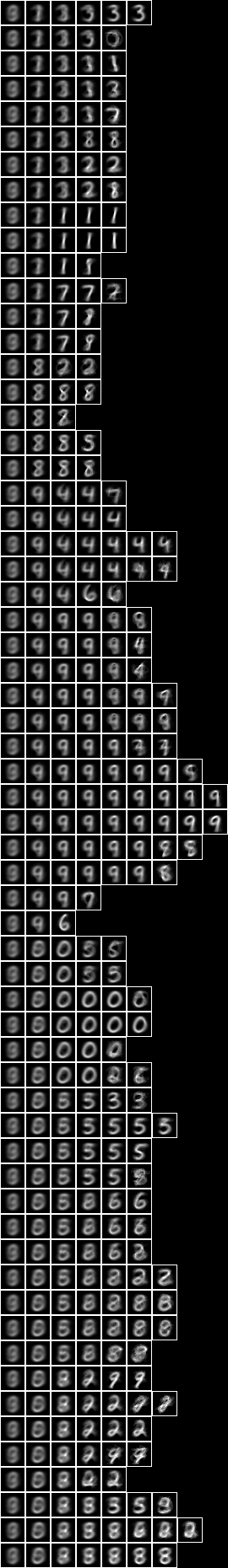Now with the fud underlying superimposed on the averaged slice,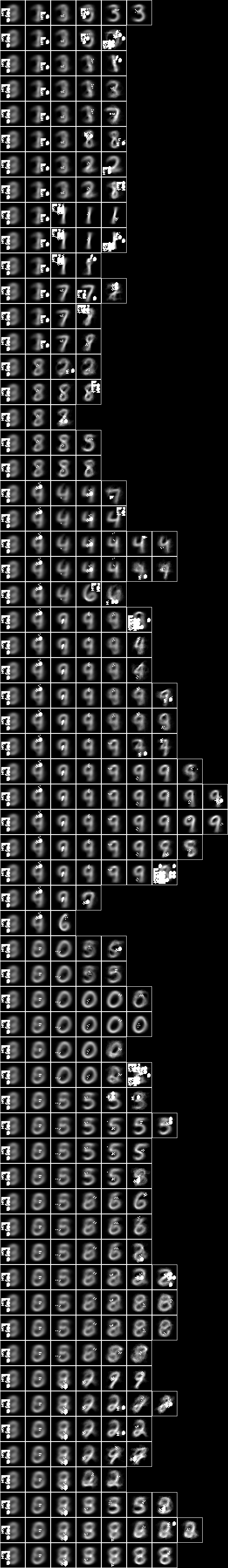If we run NIST test on the new model,

./NIST_test.exe NIST_model40 +RTS -s >NIST_test_model40.log 2>&1


we obtain the following statistics,

model: NIST_model40
selected train size: 7500
model cardinality: 2296
nullable fud cardinality: 2671
nullable fud derived cardinality: 127
nullable fud underlying cardinality: 589
ff label ent: 0.5850540595121823
test size: 1000
effective size: 1000 % 1
matches: 794


### Model 41 - Conditional level over two level over 15x15 regions

Now run the conditional entropy fud decomper over the 2-level induced model Model 25 to create Model 41,

./NIST_engine_cond.exe 2 NIST_model25 1 5 127 NIST_model41 +RTS -s >NIST_engine41.log 2>&1


This runs on on a Ubuntu 16.04 AMD EPYC 7571 cpu @ 2199.522 MHz in 11261 MB memory in 10998 seconds.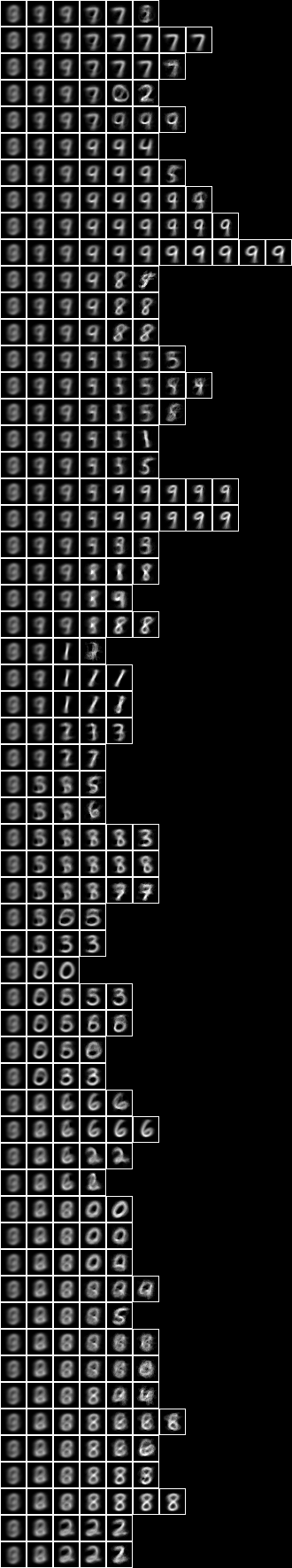Now with the fud underlying superimposed on the averaged slice,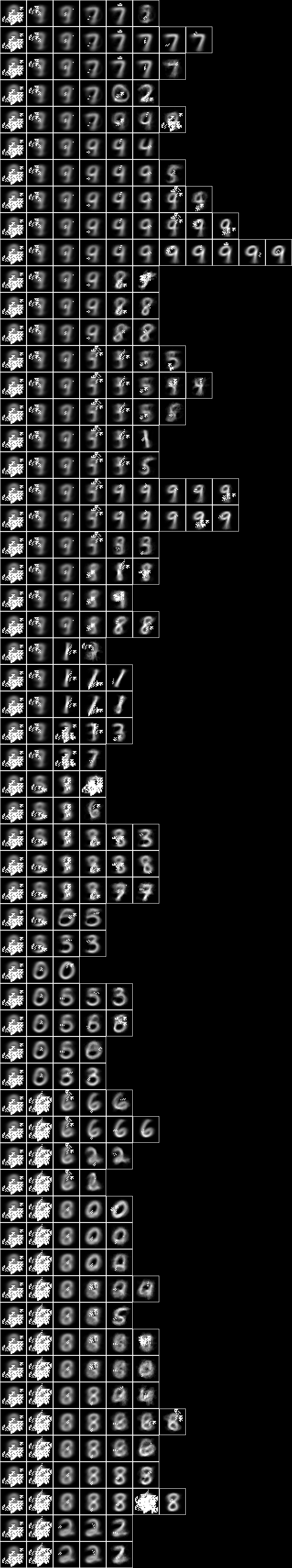If we run NIST test on the new model,

./NIST_test.exe NIST_model41 +RTS -s >NIST_test_model41.log 2>&1


we obtain the following statistics,

model: NIST_model41
selected train size: 7500
model cardinality: 1522
nullable fud cardinality: 1897
nullable fud derived cardinality: 127
nullable fud underlying cardinality: 452
ff label ent: 0.5974764620331507
test size: 1000
effective size: 994 % 1
matches: 791


### Model 42 - Conditional level over centred square regions of 11x11 pixels

Now run the conditional entropy fud decomper over the 2-level induced model Model 26 to create Model 42,

./NIST_engine_cond.exe 2 NIST_model26 1 5 127 NIST_model42 +RTS -s >NIST_engine42.log 2>&1


This runs on on a Ubuntu 16.04 AMD EPYC 7571 cpu @ 2199.522 MHz in 15636 MB memory in 17241 seconds.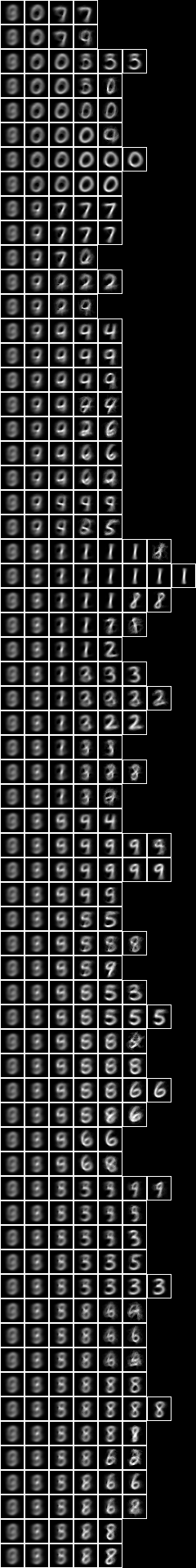Now with the fud underlying superimposed on the averaged slice,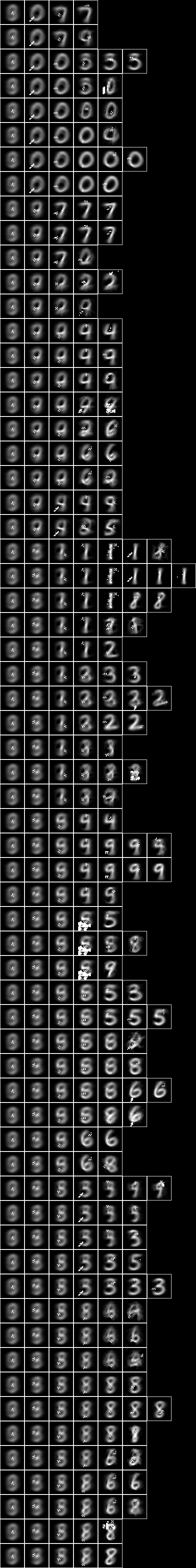If we run NIST test on the new model,

./NIST_test.exe NIST_model42 +RTS -s >NIST_test_model42.log 2>&1


we obtain the following statistics,

model: NIST_model42
selected train size: 7500
model cardinality: 835
nullable fud cardinality: 1211
nullable fud derived cardinality: 127
nullable fud underlying cardinality: 311
ff label ent: 0.6526213410903168
test size: 1000
effective size: 1000 % 1
matches: 786


top